# On Wijsman asymptotically lacunary I-statistical equivalence of weight g of sequence of sets

### Full PDFcreative_2019_28_1_61_68

This paper presents the following definition which is a natural combination of the definitions of asymptotically equivalence,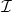-convergence, statistical limit, lacunary sequence, and Wijsman convergence of weight; where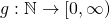is a function satisfying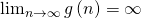and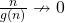as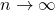for sequence of sets. Let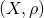be a metric space,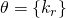be a lacunary sequence and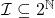be an admissible ideal. For any non-empty closed subsets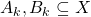such that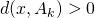and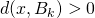for each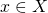, we say that the sequences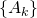and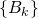are Wijsman-asymptotically lacunary statistical equivalent of multiple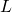of weightif for every,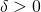and for each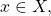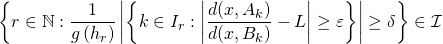(denoted by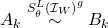. We mainly investigate their relationship and also make some observations about these classes.